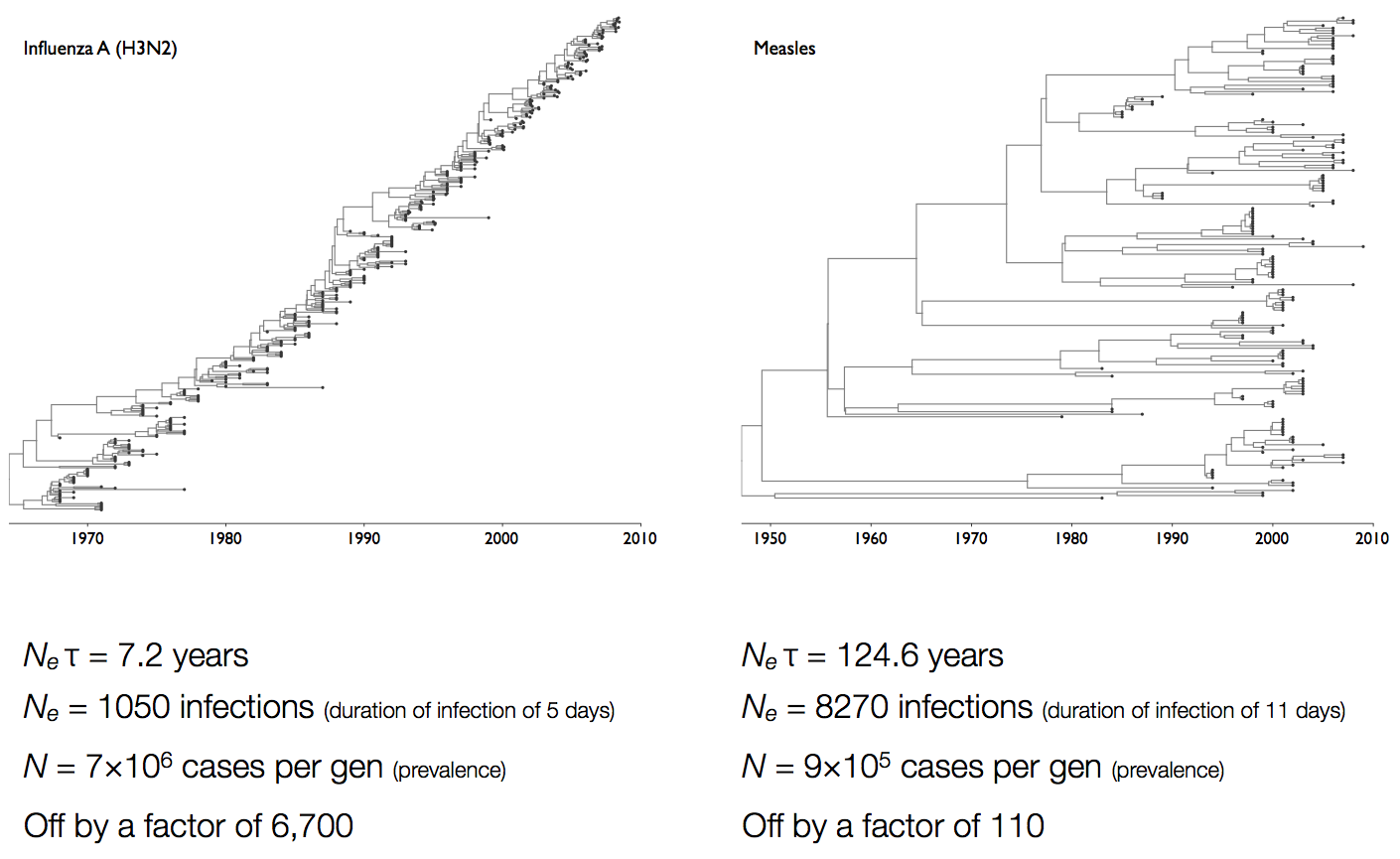## Molecular clocks and the coalescent

Trevor Bedford (@trvrb)
May 26, 2020
GS541 Introduction to Computational Molecular Biology

## Molecular clocks and dated phylogenies

### Mutations tend to accumulate in a clock-like fashion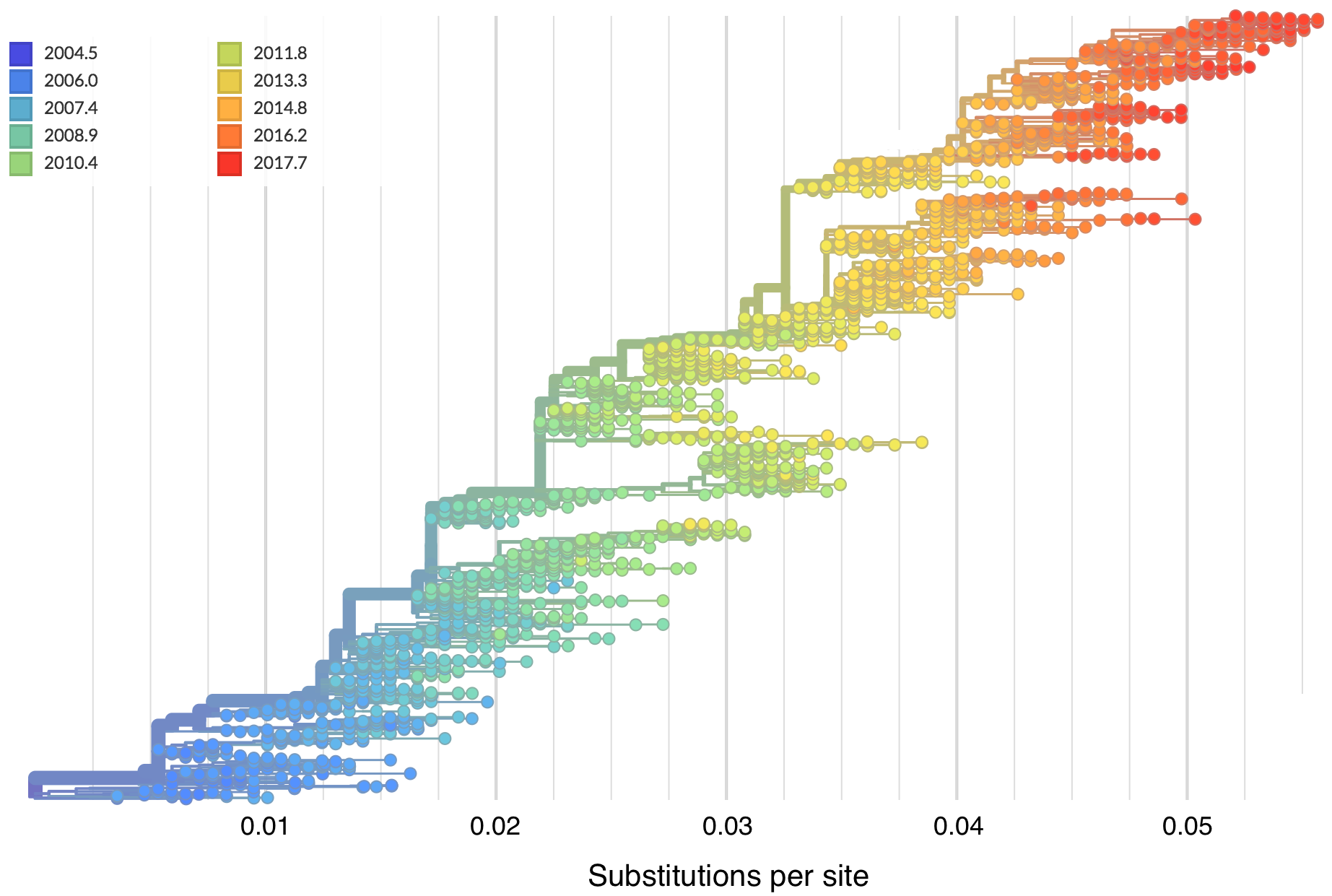### "Root-to-tip" plots show temporal signal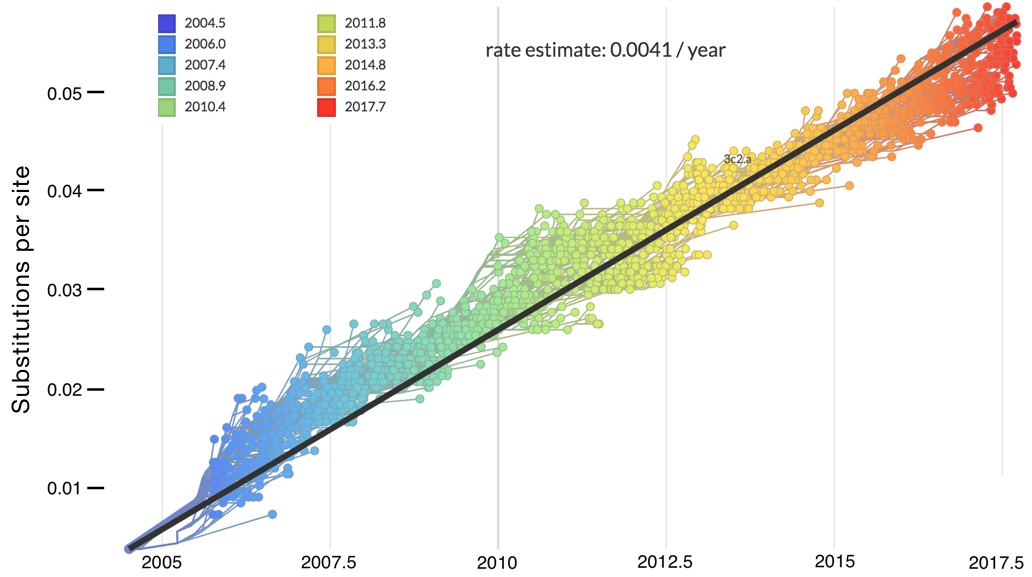### Allows conversion between branch length and time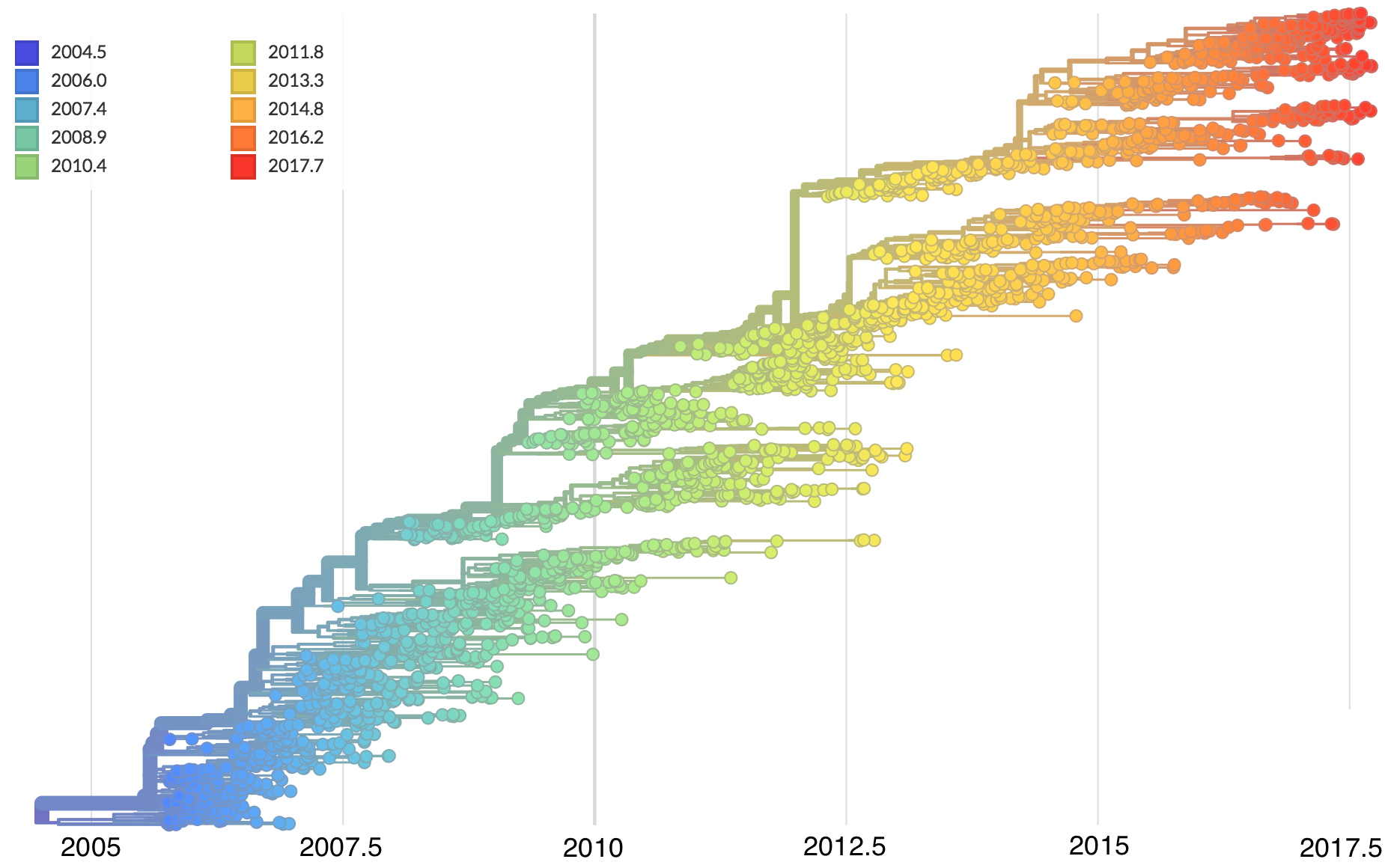### Dated phylogenies provide real-world context### Conversion between mutations and time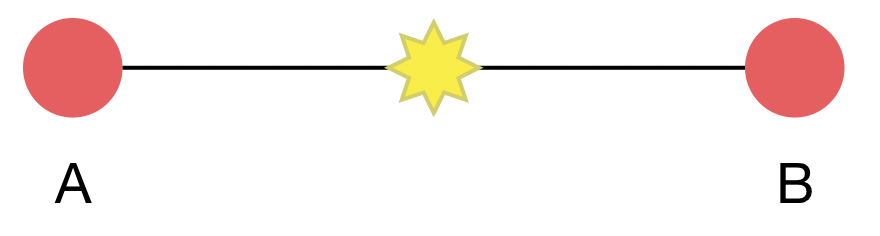Taxa A and B are separated by 1 mutation, where divergence is low relative to alignment length, ie 1 mutation in a 30 kb genome. Evolutionary rate μ = 2 mutations per month.

This is a Poisson process in which k events are observed in an unknown amount of time t, but with known rate μ. $k \sim Poisson(\mu \, t)$

### Conversion between mutations and timeWith $k = 1$ and $\mu = 2$, we have $\mathrm{Pr}(k|t) = 2 \, t \, e^{-2 \, t}$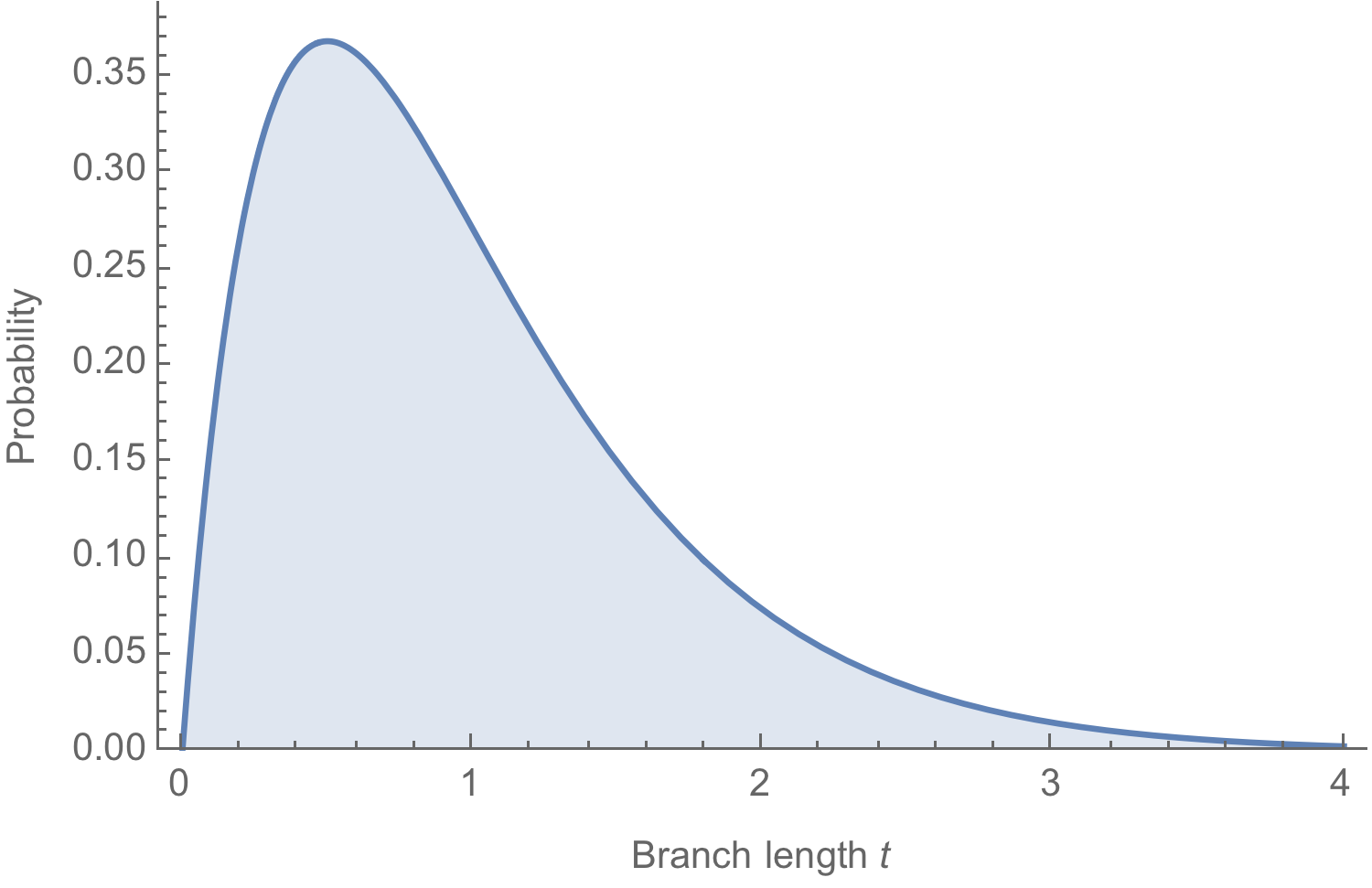### Conversion between mutations and time

Likelihoods multiplied across branches and μ estimated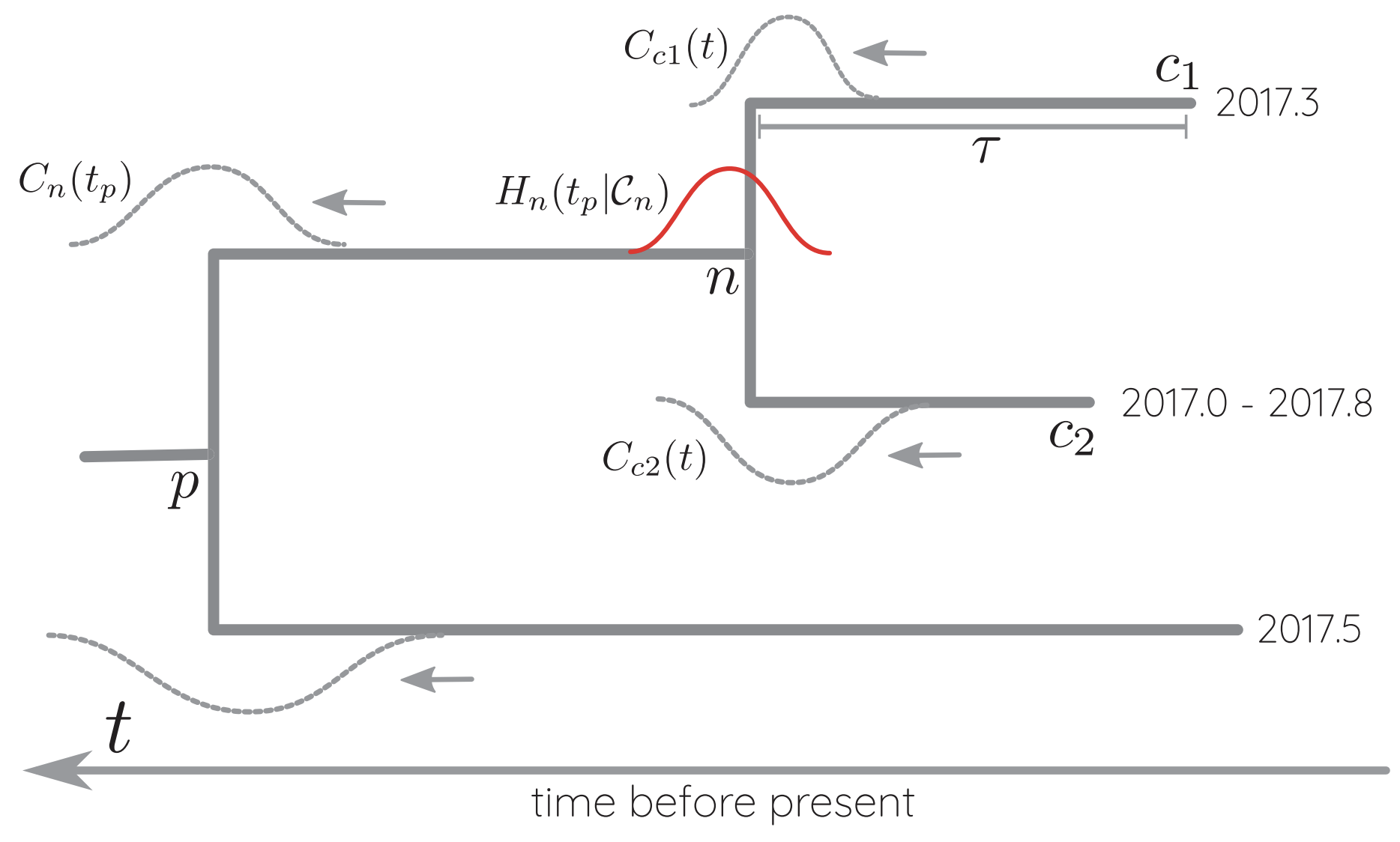Approaches:

## The coalescent

### Wright-Fisher process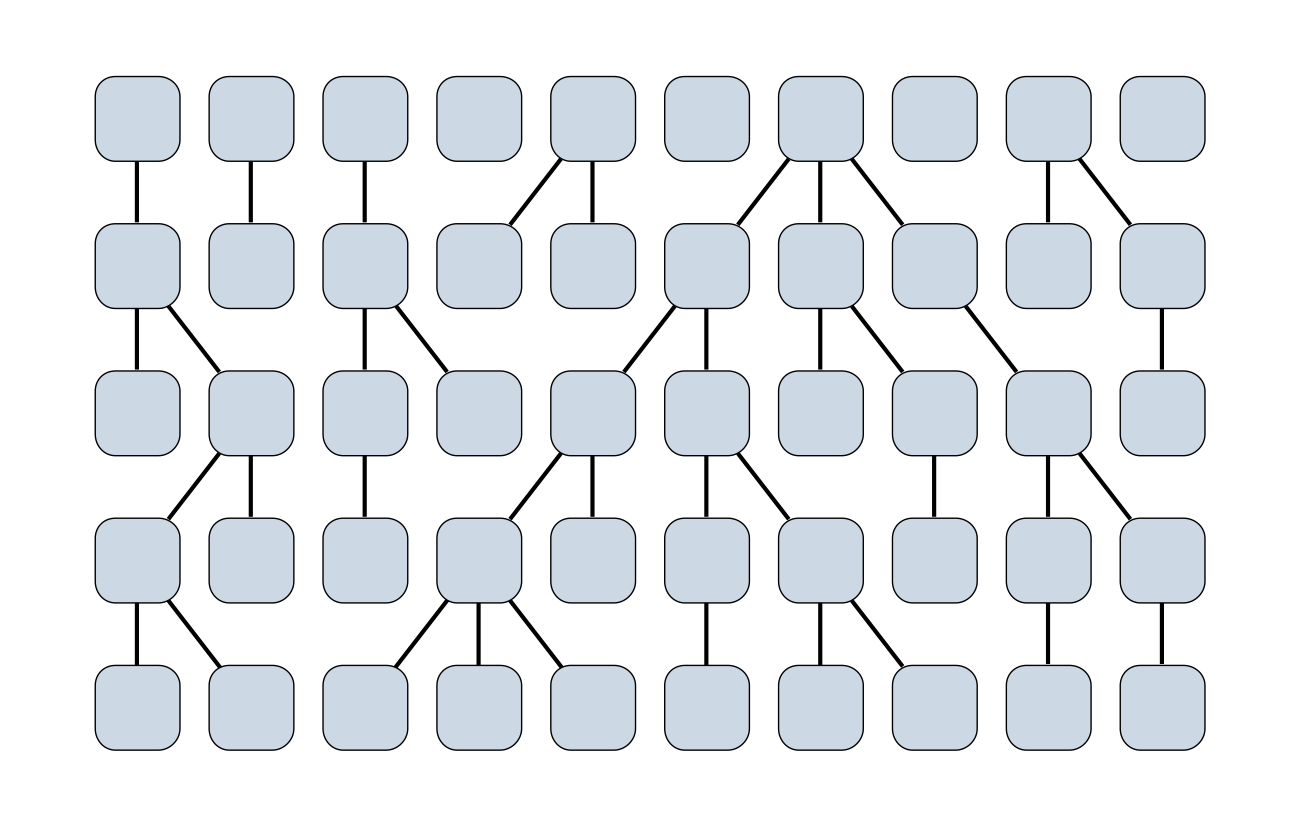### Sample some individuals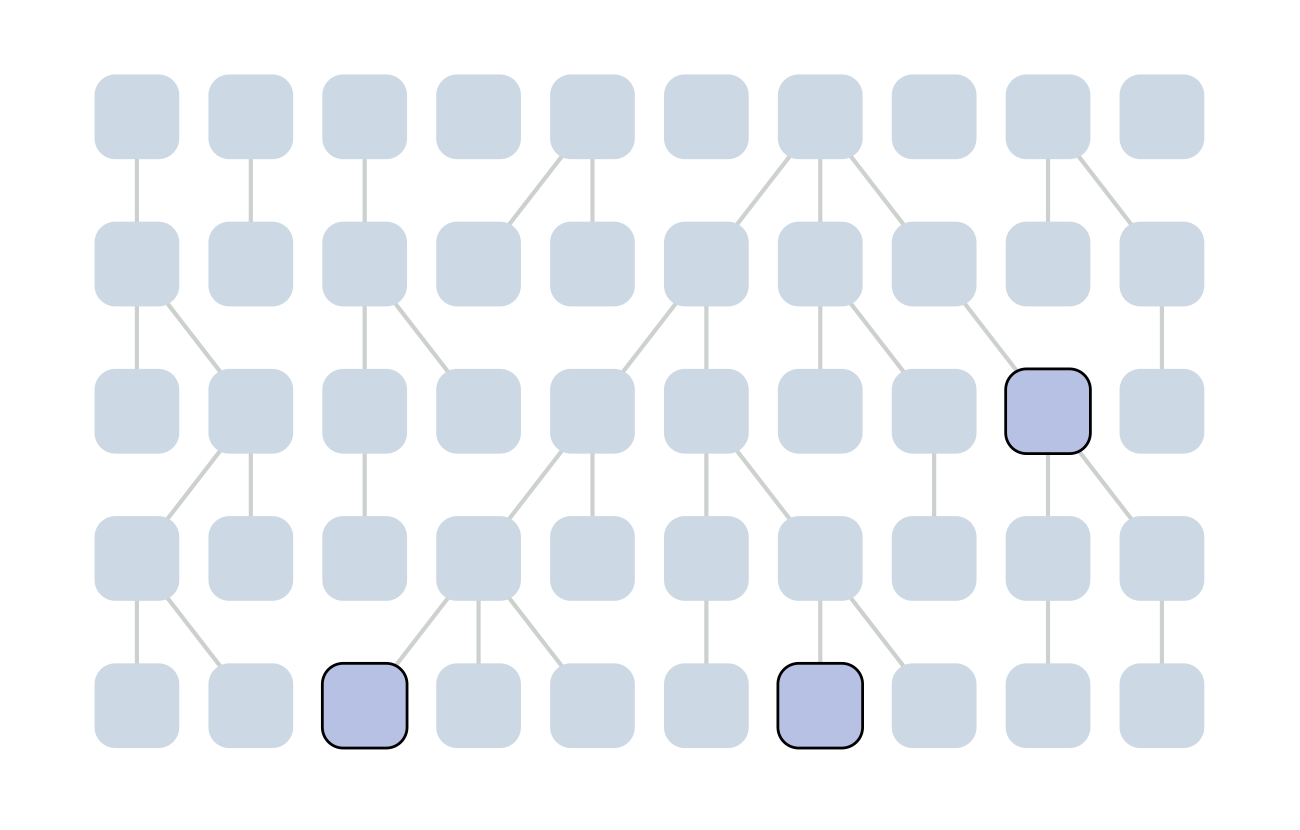### Each generation coalescence may occur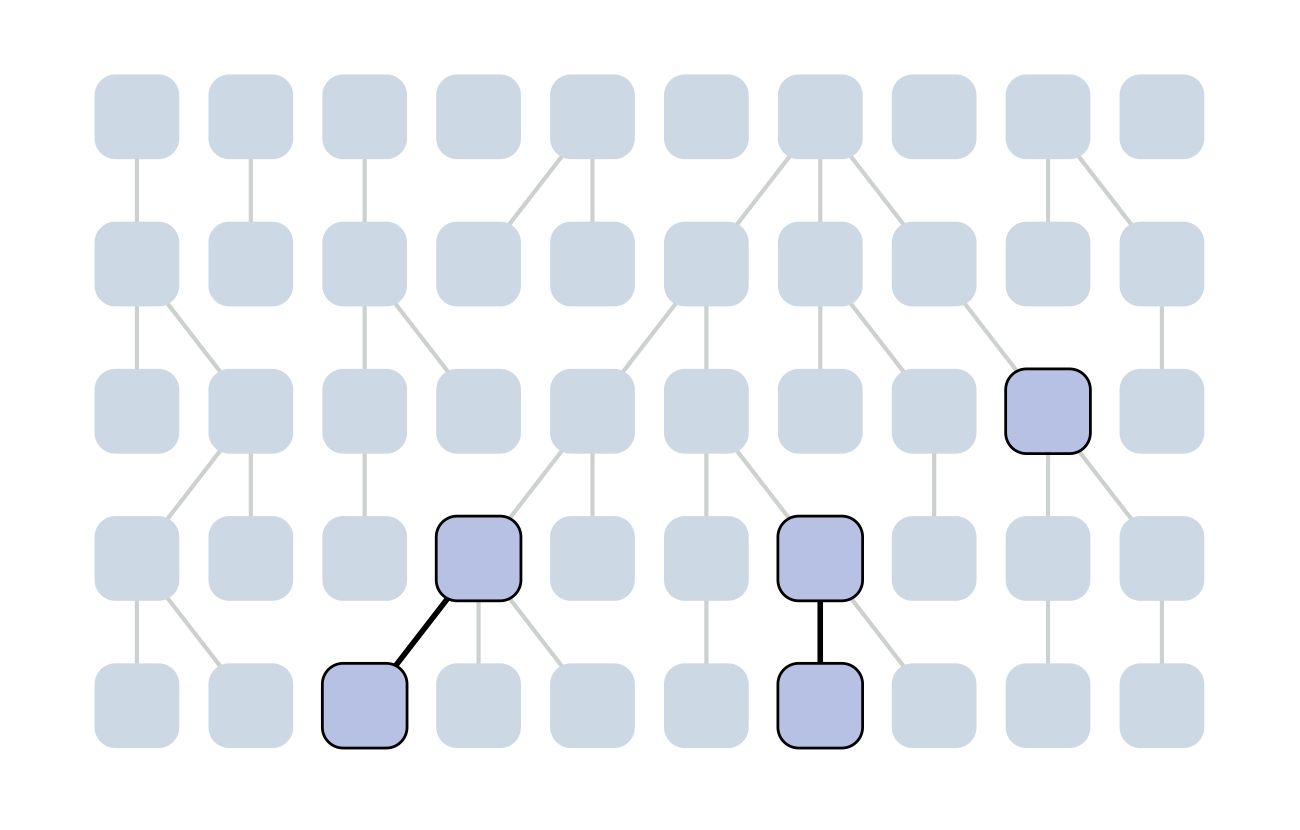### Each generation coalescence may occur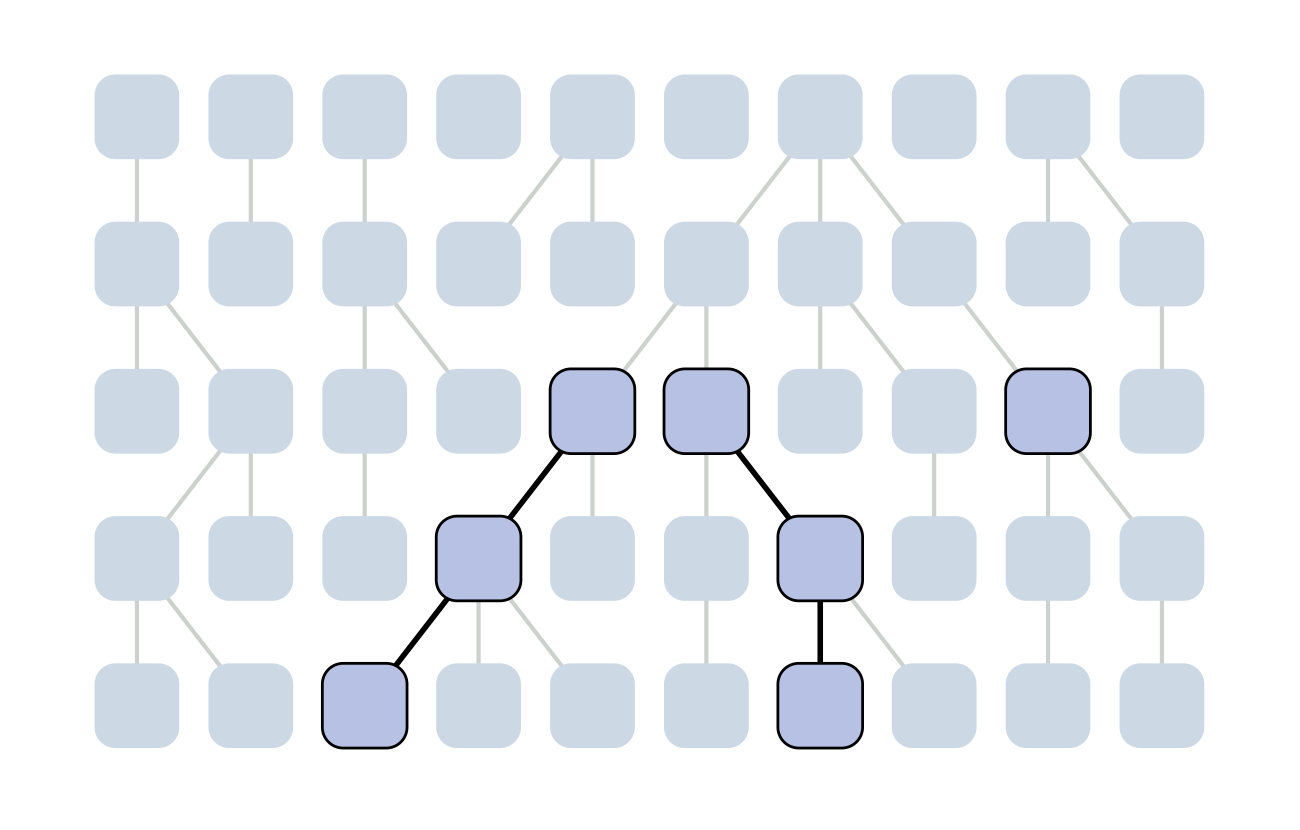### Each generation coalescence may occur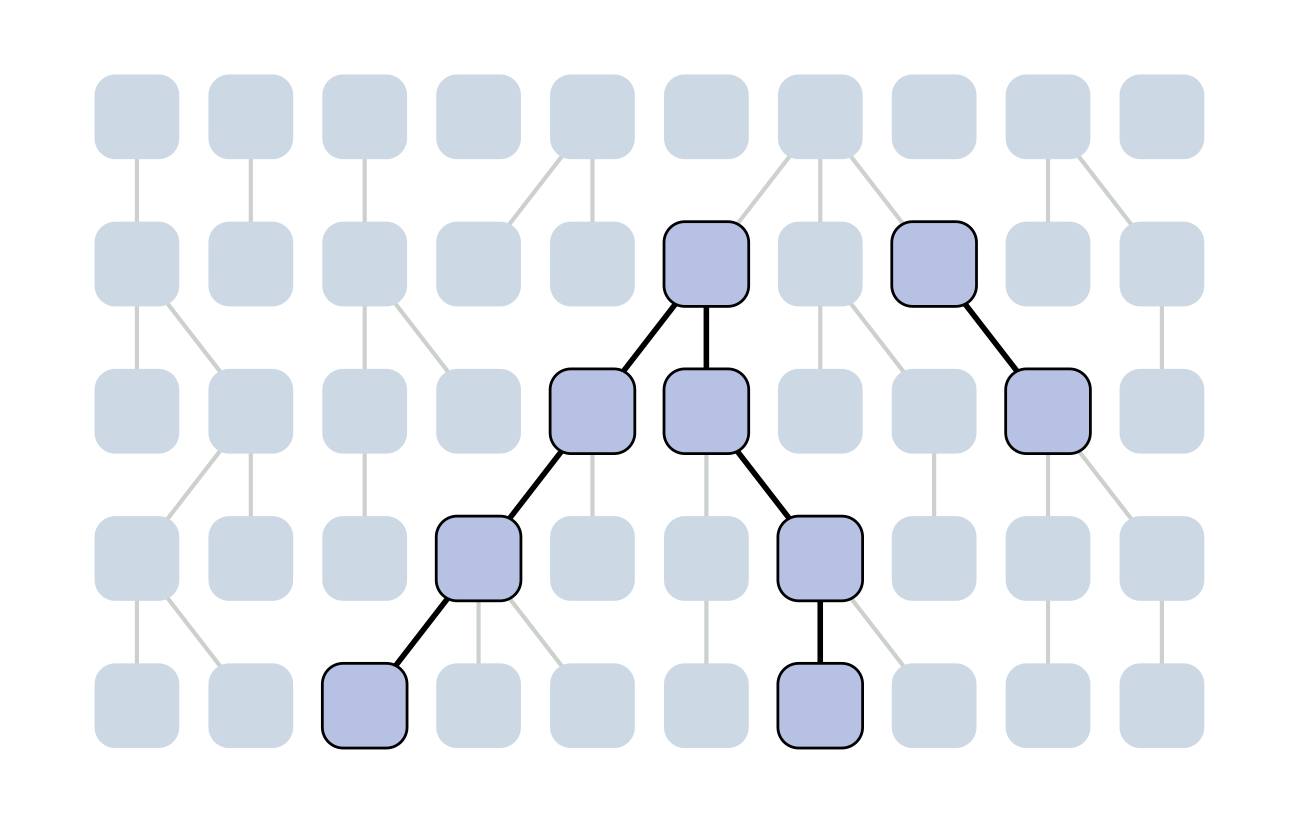### Each generation coalescence may occur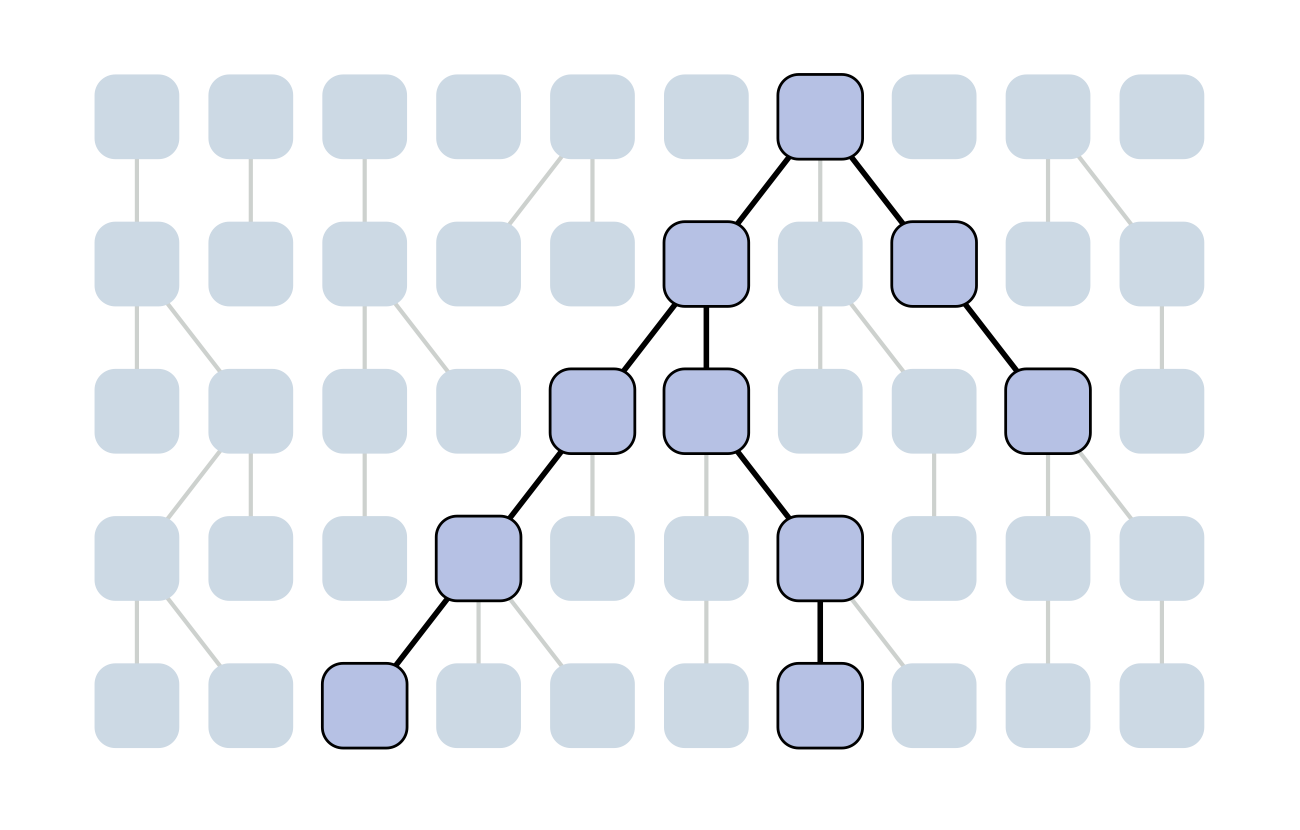### Set of coalescent intervals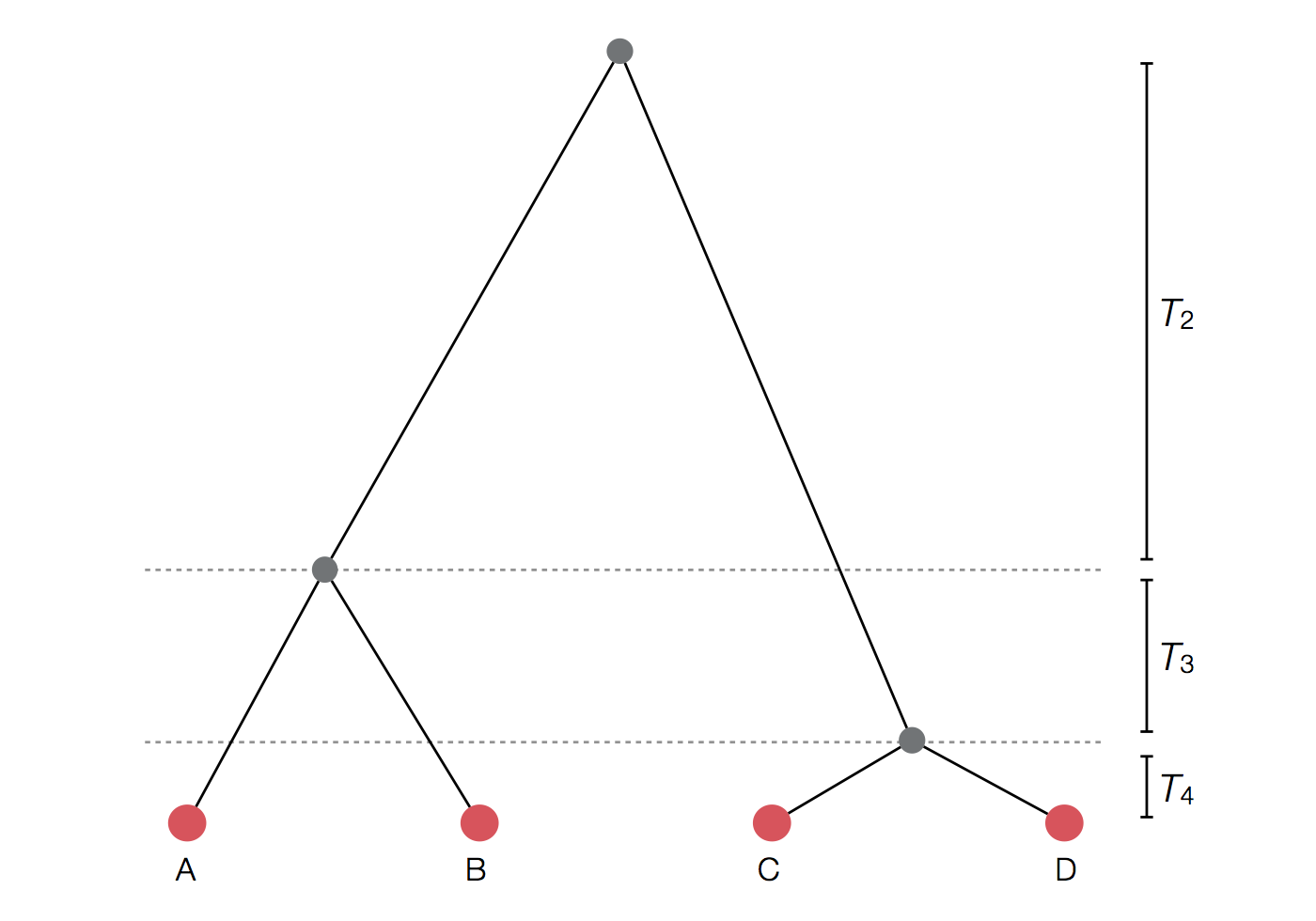### Per-generation probability of coalescence

$$\mathrm{Pr}(\mathrm{coal} \, | \, i=2) = \frac{1}{N}$$

Probability of first lineage picking an arbitrary parent is 1, while the probability of the 2nd lineage picking the same parent is $\frac{1}{N}$.

Probability of coalescence scales inversely with population size.

### Per-generation probability of coalescence

$$\mathrm{Pr}(\mathrm{coal}) = \binom{i}{2} \frac{1}{N} = \frac{i(i-1)}{2N}$$

There are $\binom{i}{2}$ ways pairs of lineages can pick the same parent.

Probability of coalescence scales quadratically with lineage count.

### Expected waiting time to coalescence

$$\mathrm{E}[T_i] = \frac{2N}{i(i-1)}$$

This is a geometric distribution. If each generation there is a $\frac{1}{x}$ probability of an event occurring, we expect to wait $x$ generations for the event to occur.

### Continuous time limit

With per-generation probability of an event $\frac{1}{x}$ small, but many generations, then the discrete time geometric distribution approximates to a continuous time exponential distribution. Thus, we assume $T_i$ to be exponentially distributed with mean

$$\mathrm{E}[T_i] = \frac{2N}{i(i-1)}.$$

### Set of coalescent intervals with waiting times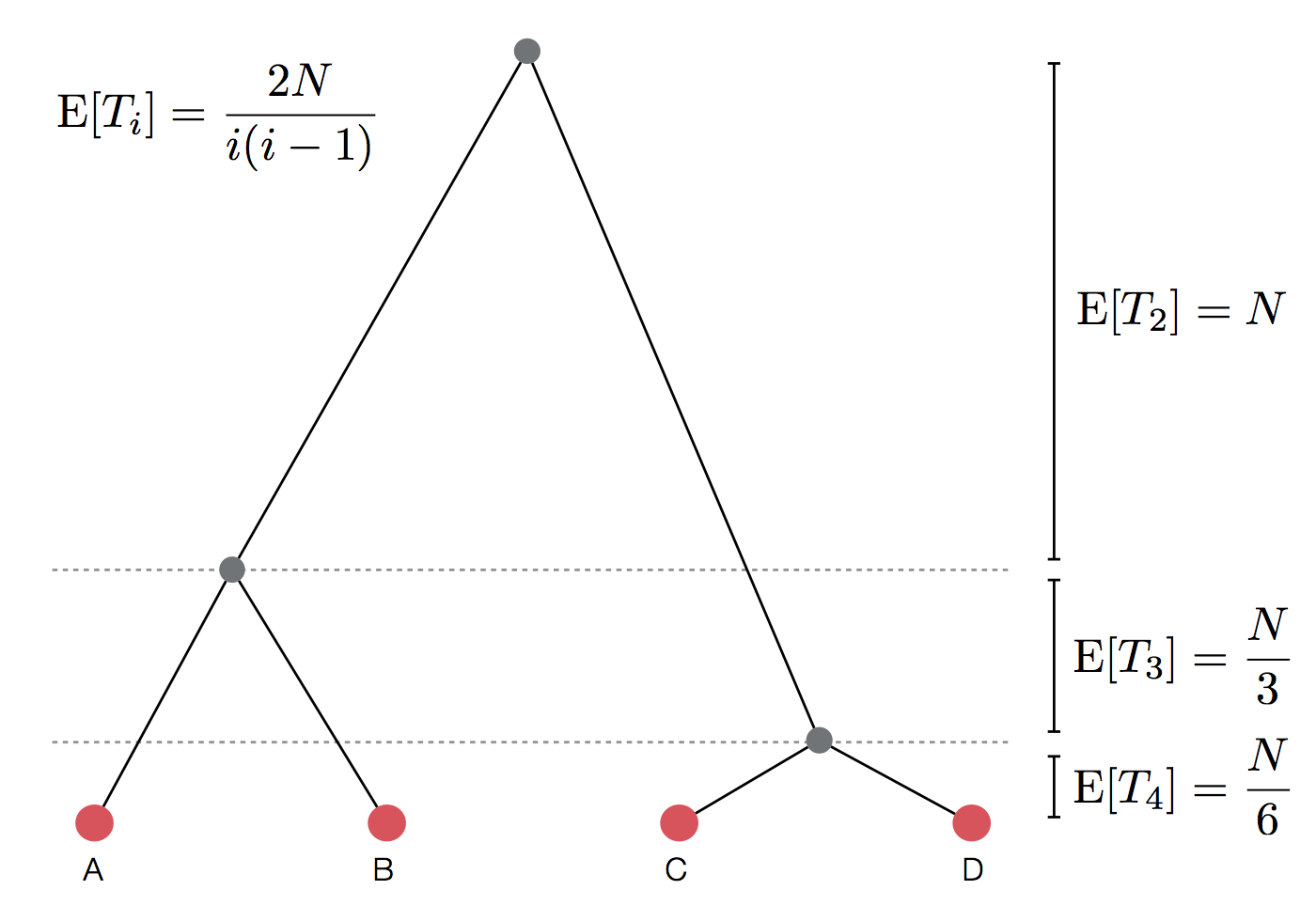### Gives coalescent trees their distinctive shape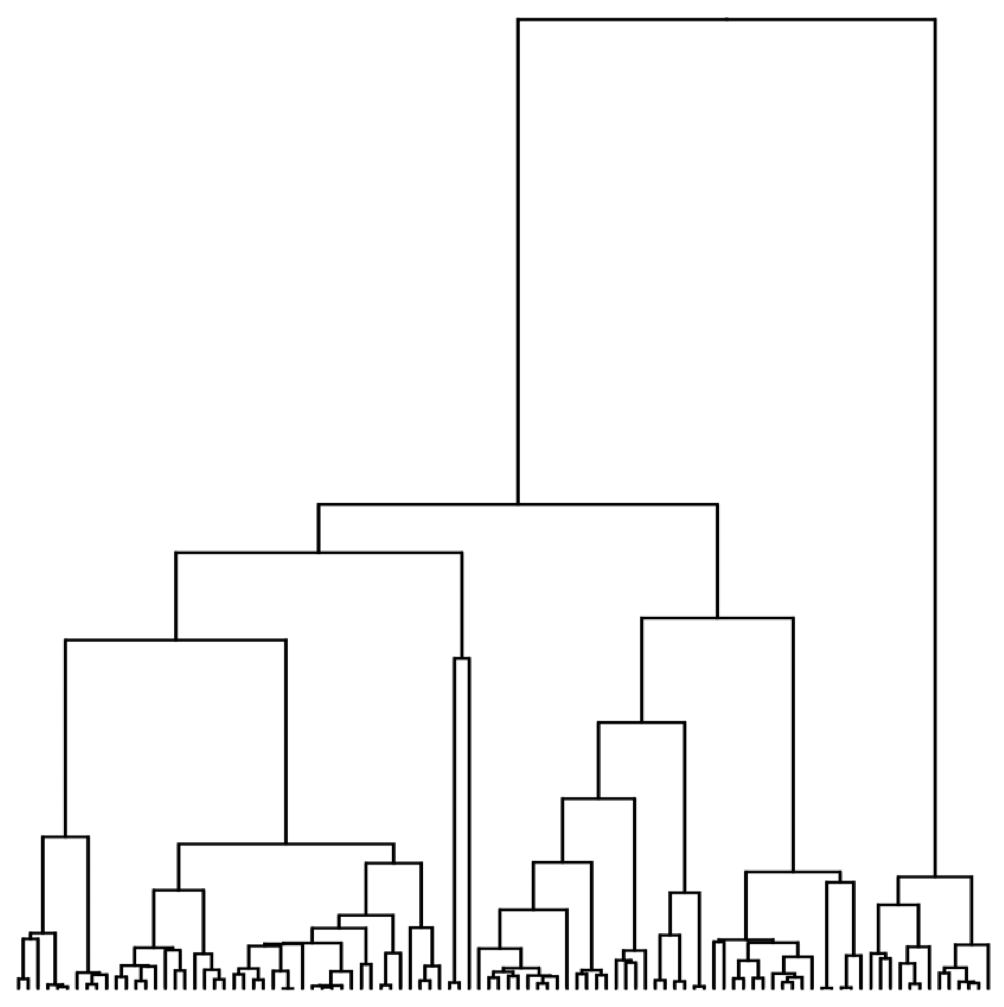### Rate of coalescence scales with population size N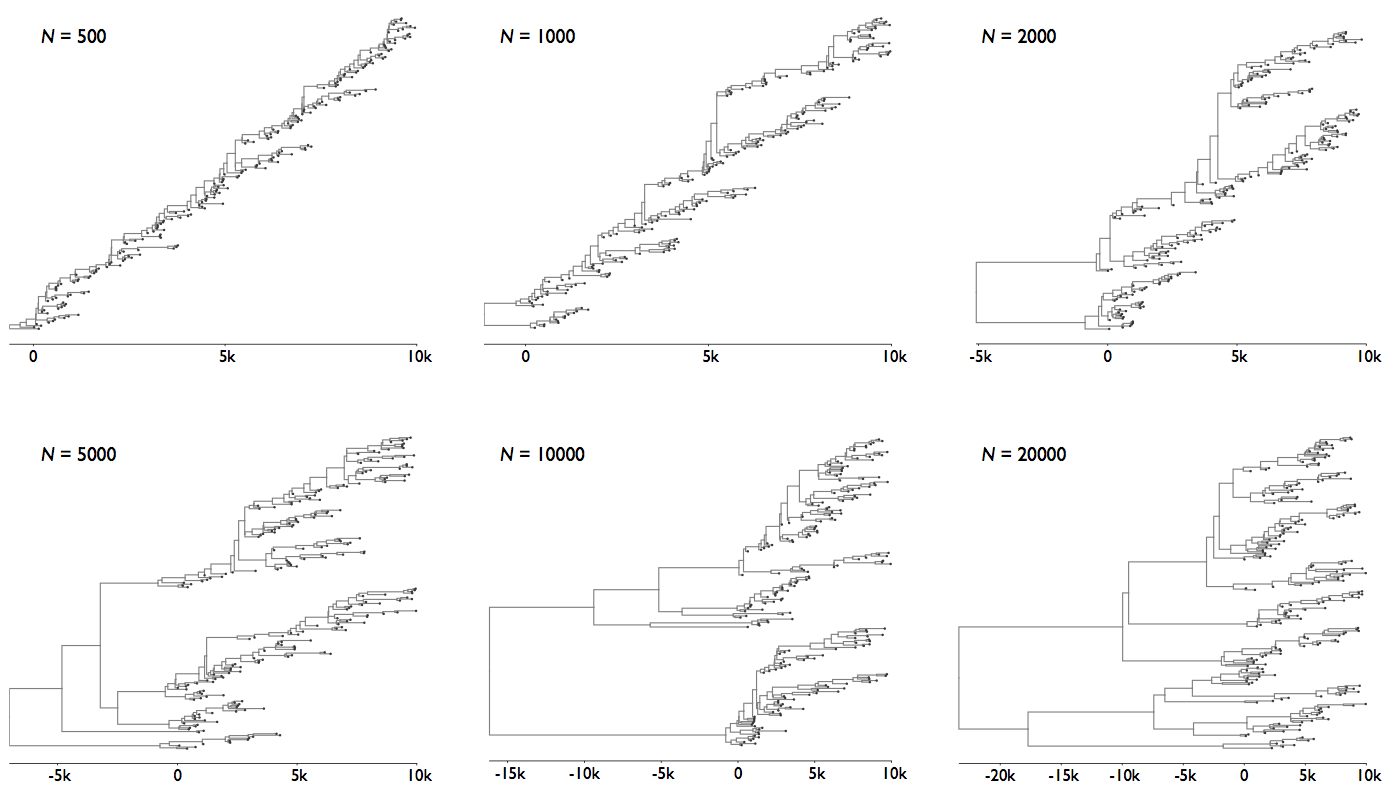## Visualization of the coalescent process

### Pairwise genetic diversity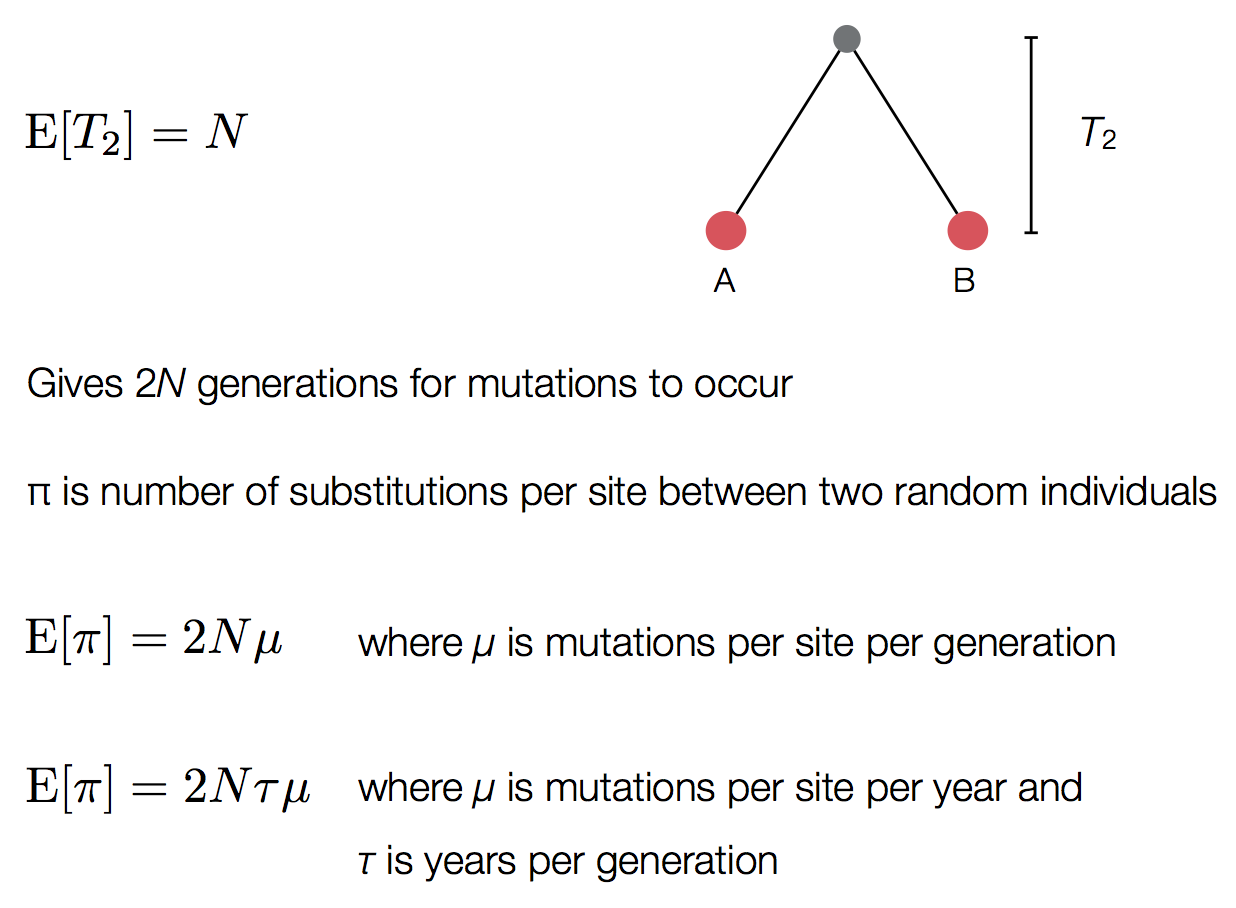### Time to the most recent common ancestor (TMRCA)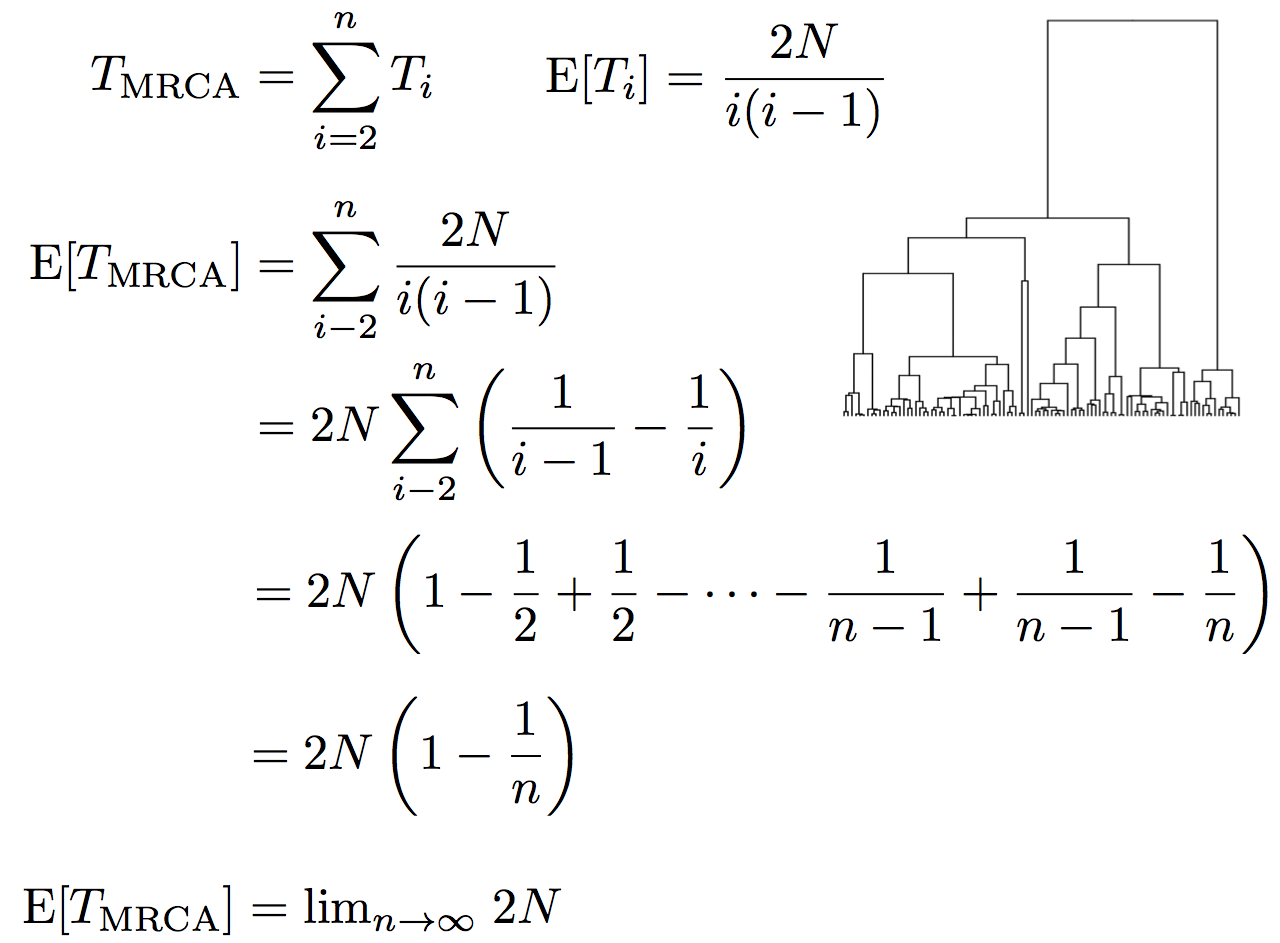## Coalescence patterns can estimate population growth/decline

### Changing population size alters coalescent rate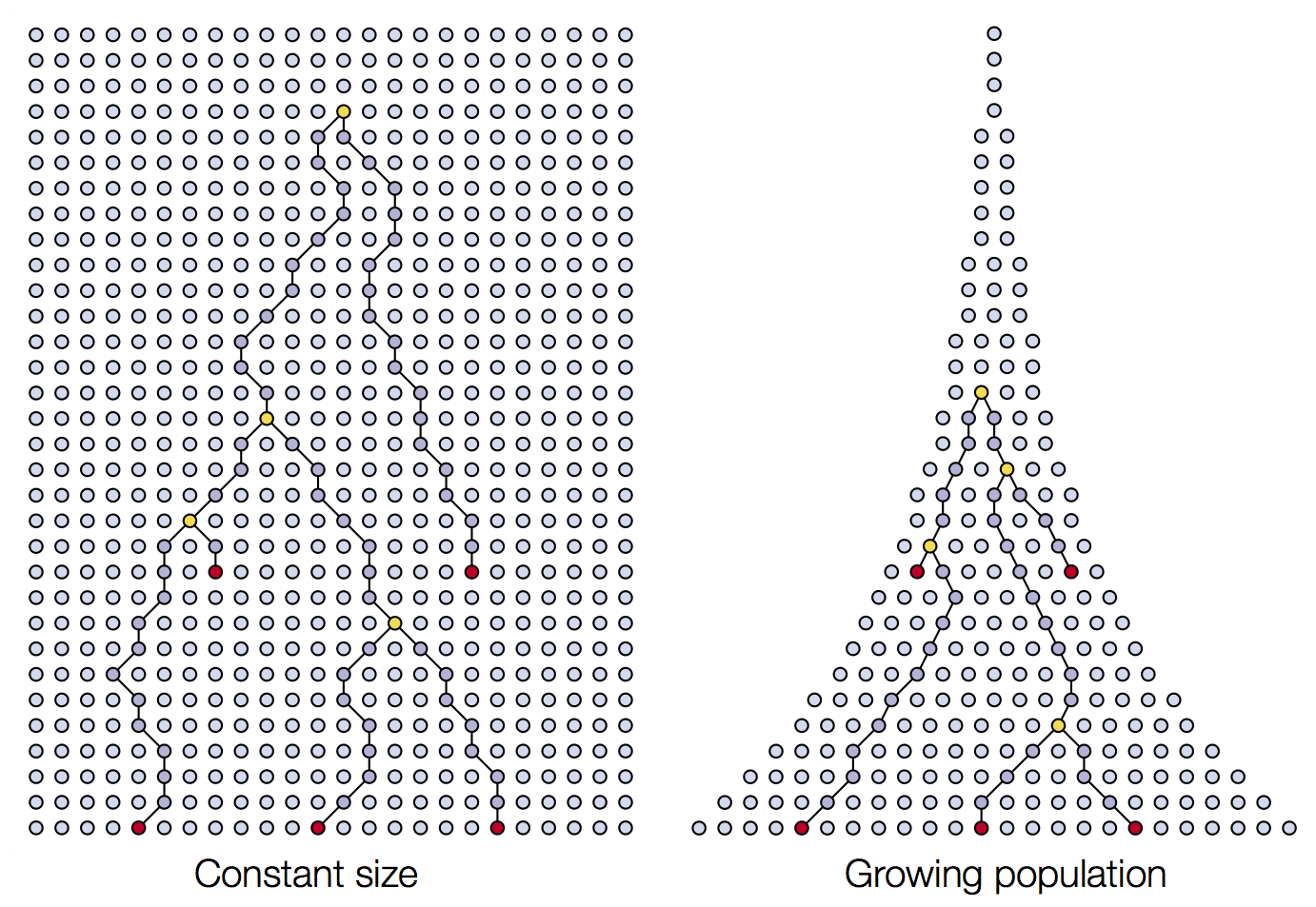### Changing population size alters coalescent rate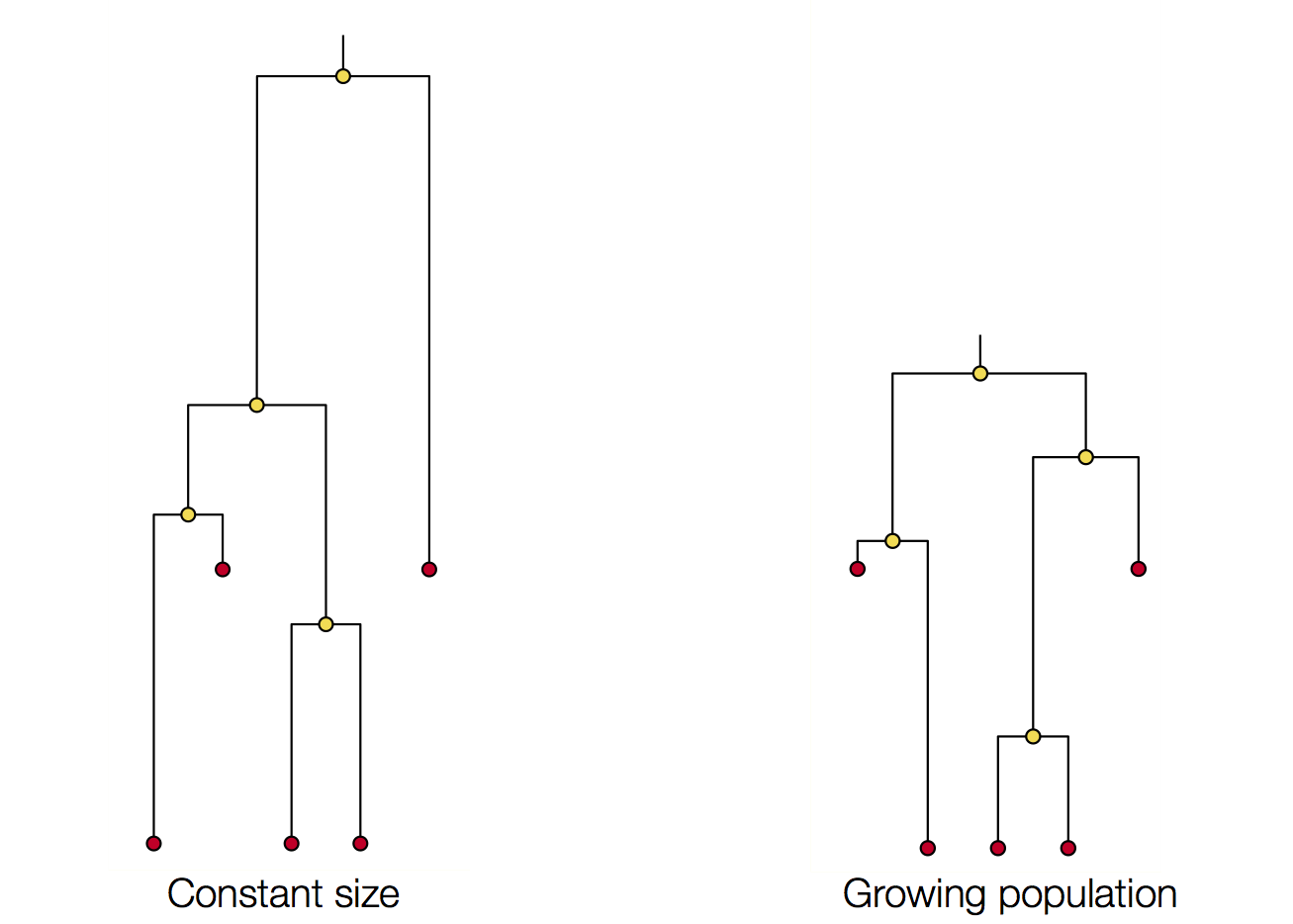### Tajima's D statistic summarizes deviation from neutrality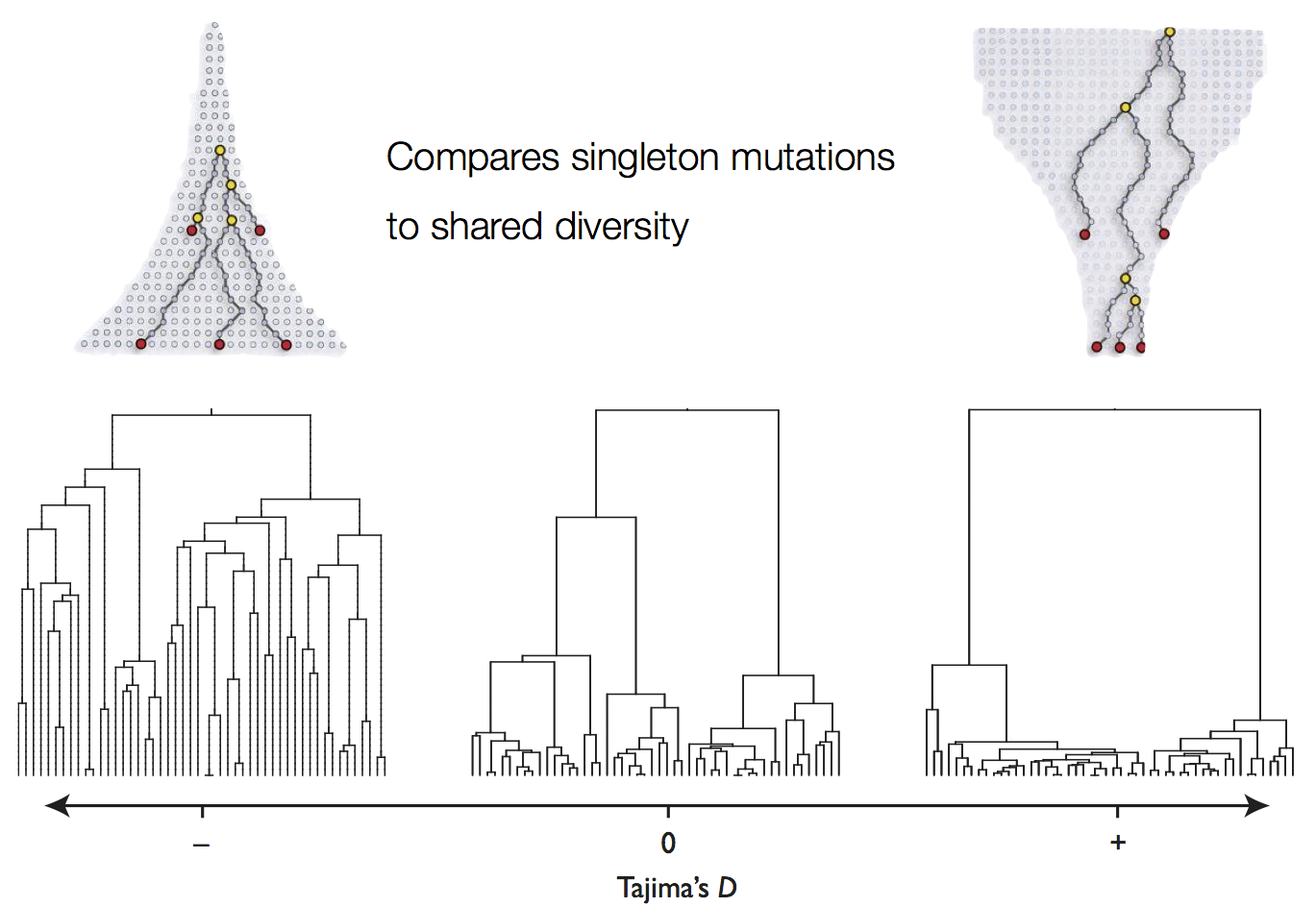### 'Skyline' is flexible demographic model that estimates windows of coalescent rate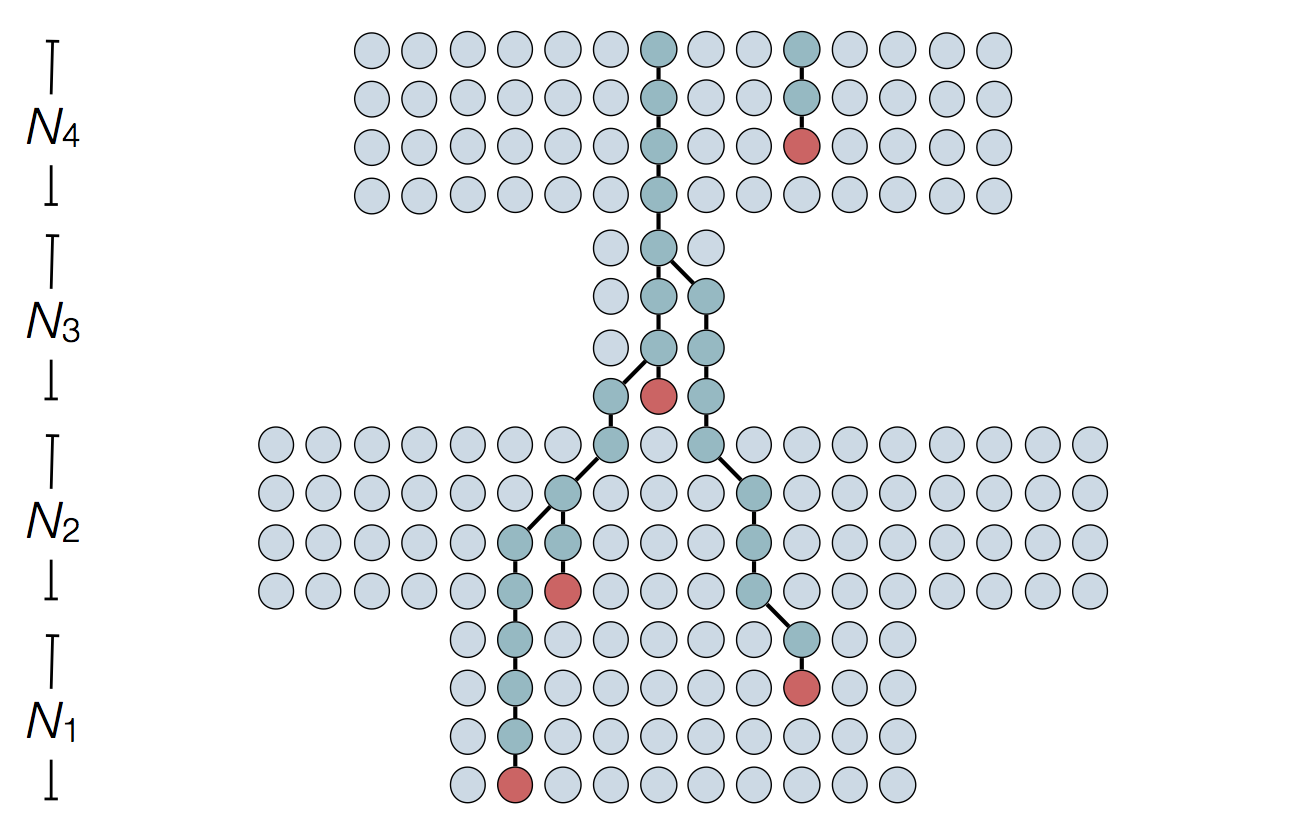### Skyline model shows population growth in HCV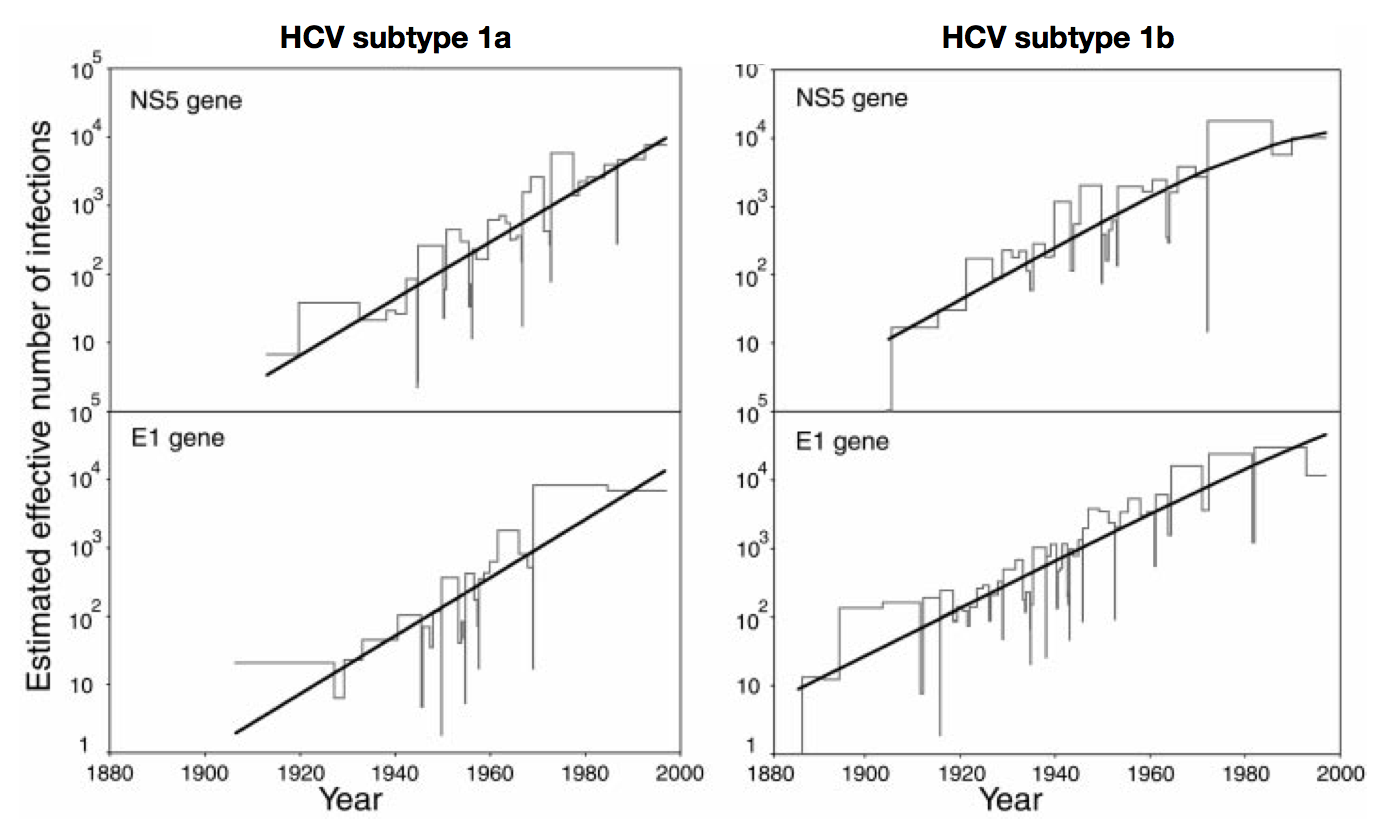### Skyline model shows seasonality in flu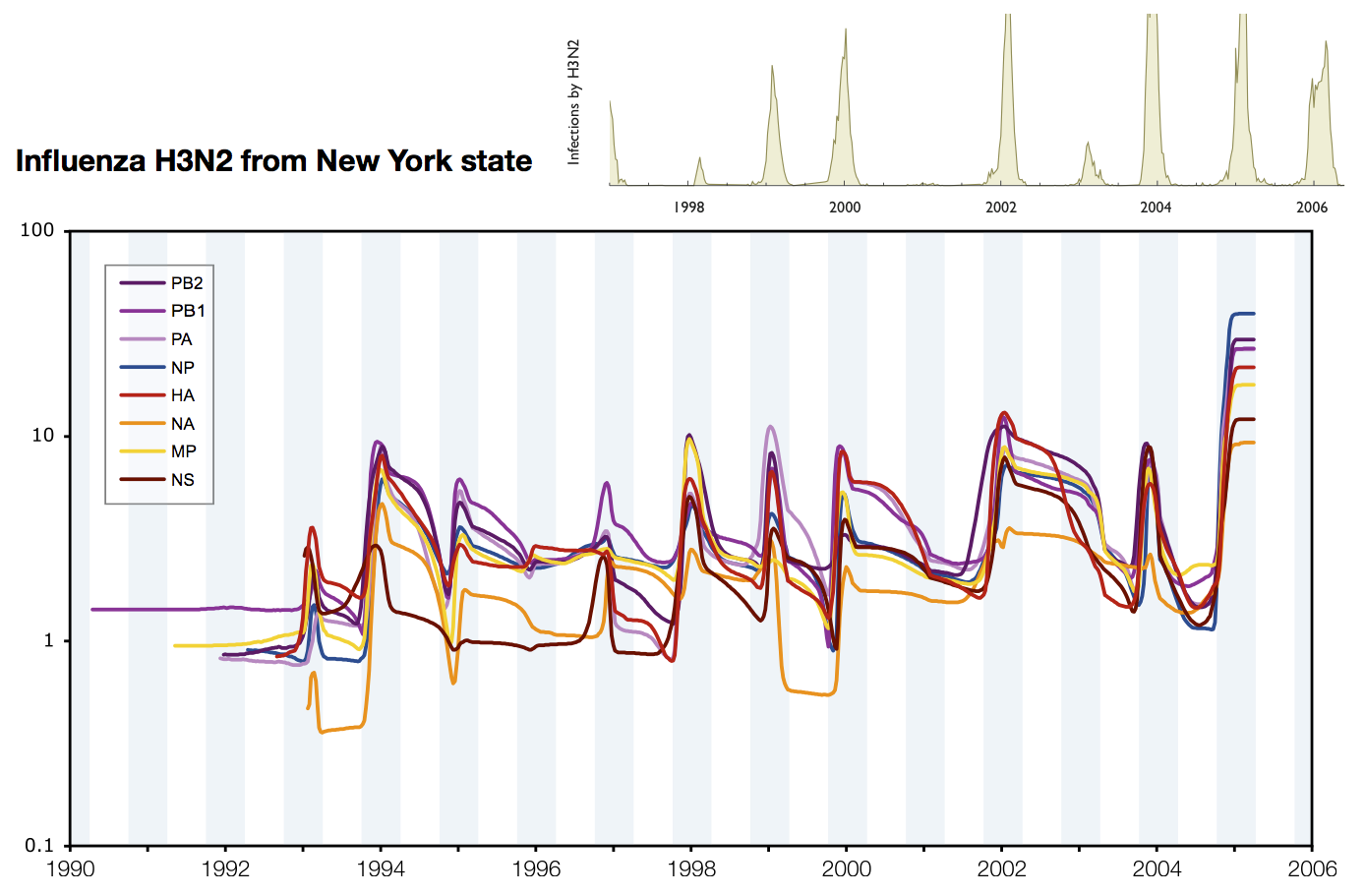### Interpreting rate of coalescence

These approaches directly estimate the pairwise rate of coalescence $\lambda$, which is measured in terms of events per year. Thus, the timescale of coalescence $\frac{1}{\lambda}$ is measured as the expected waiting time in years for two lineages to find a common ancestor.

### Interpreting rate of coalescence

The timescale of coalescence $\frac{1}{\lambda}$ is equal to $N_e\tau$, where $N_e$ is measured in generations and $\tau$ is measured in years per generation. $\tau$ acts to rescale time from generations to years.

### Example tree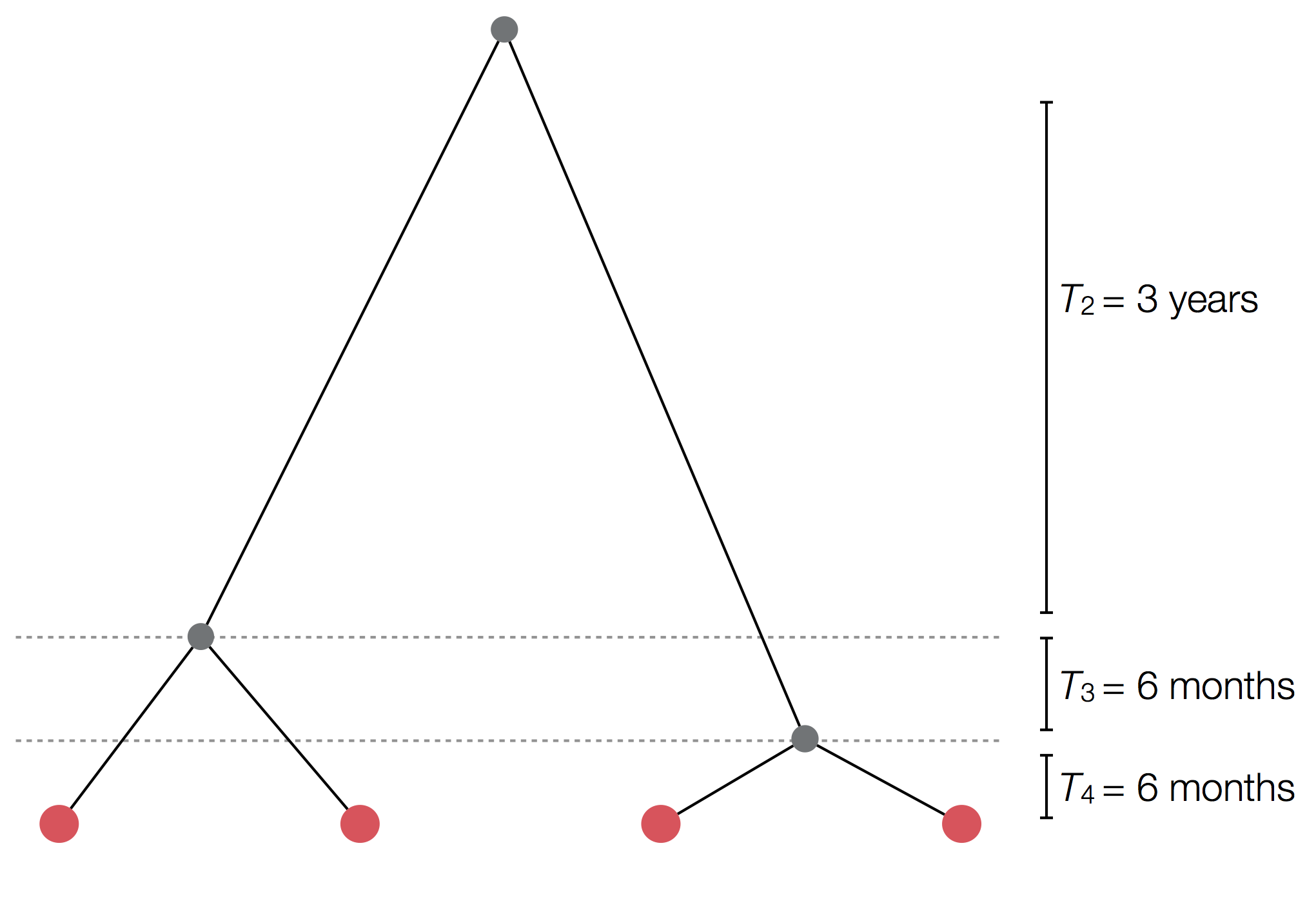### Example skyline plot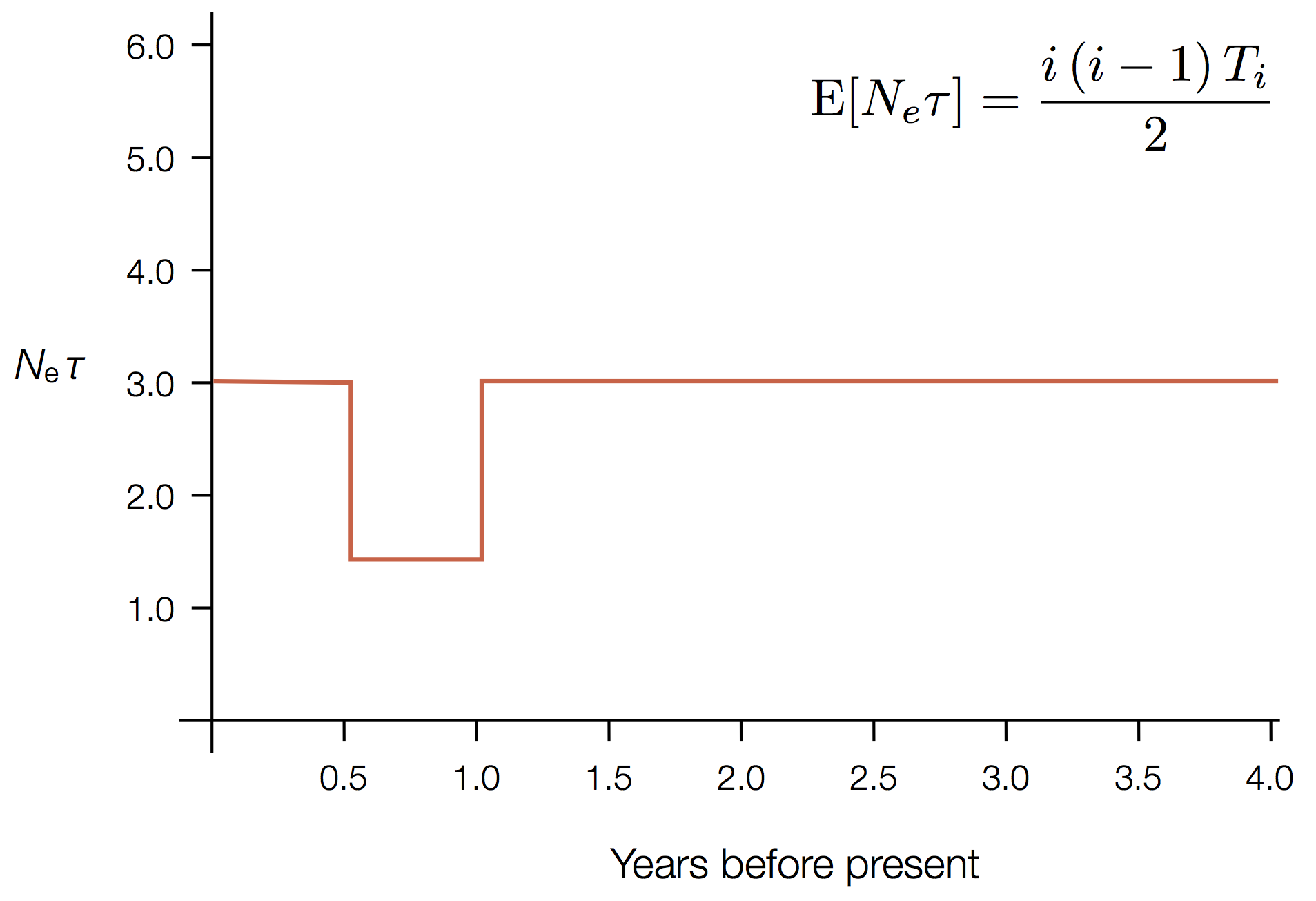### Exercise: plot skyline from tree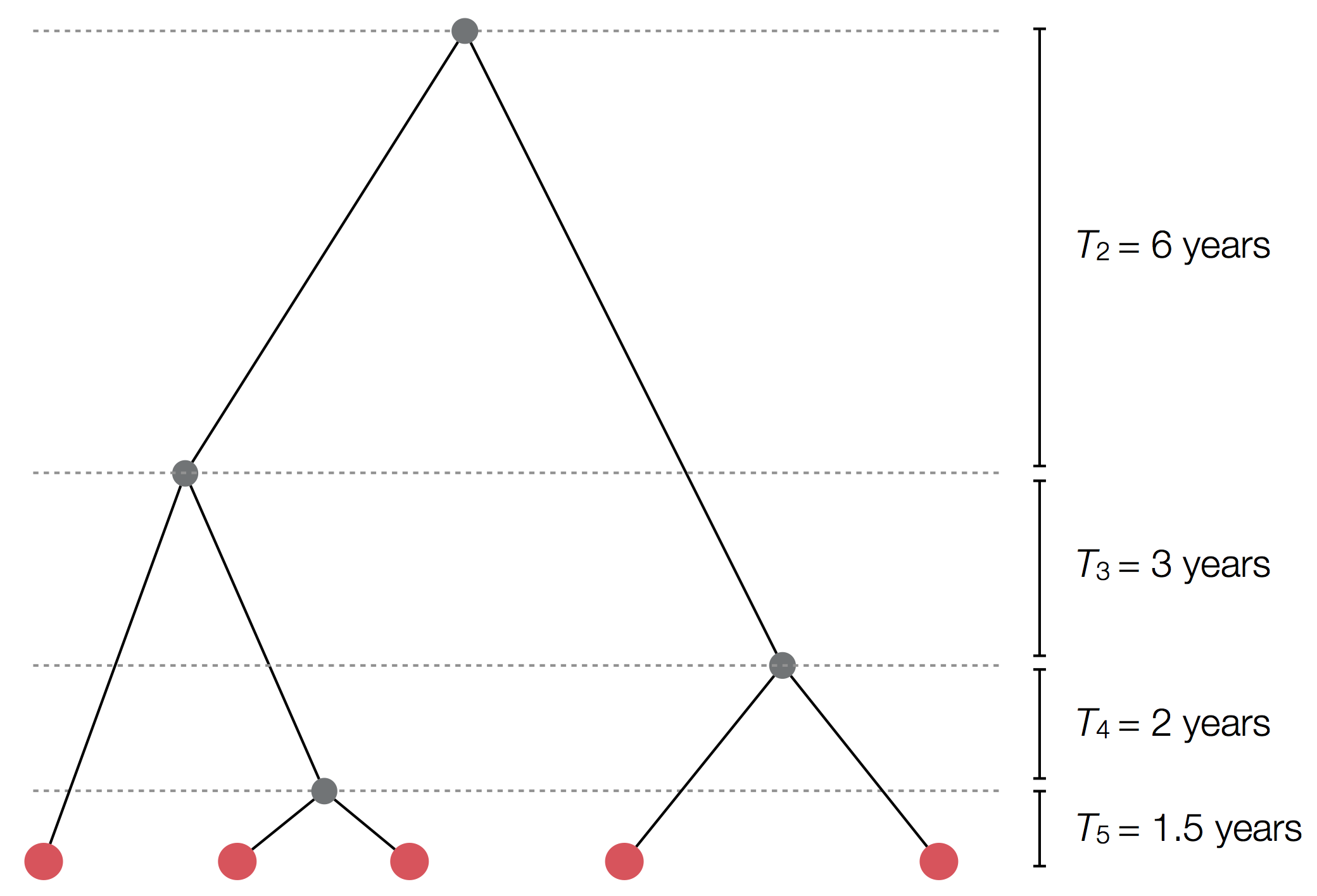### Influenza phylogeny and effective number of infections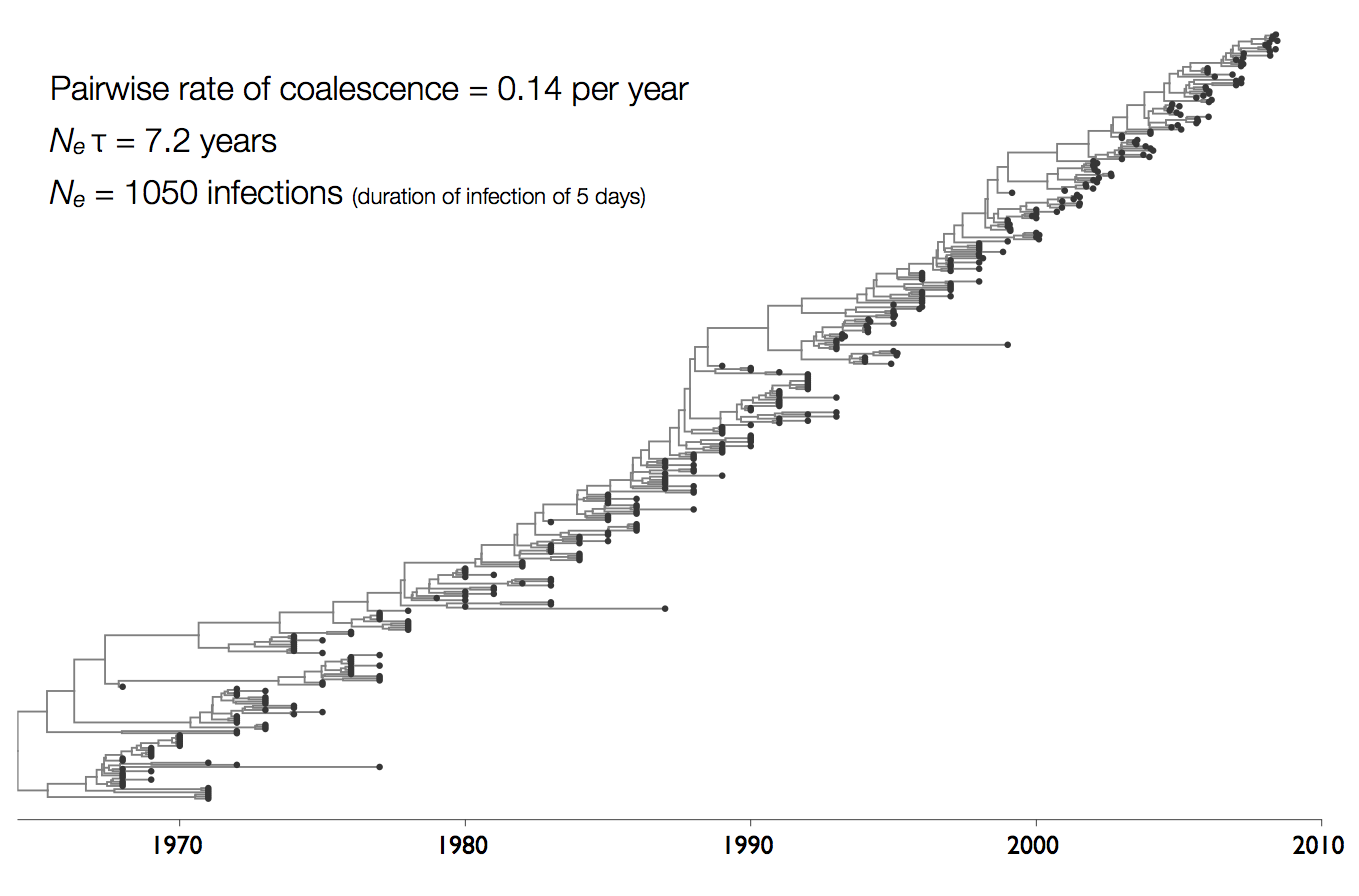### Coalescent rates in flu and measles